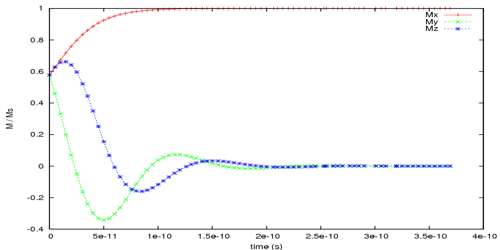# Temporal Periodicity

Temporal periodicity

If the time period of periodic motion is with respect to time, then it is called temporal periodicity. For example, the earth rotating round the sun comes to a particular place in 365 days; again, the moon takes 30 days for a completes rotation around the earth. Rotation of satellites around the earth etc. is an example of temporal periodicity. Periodic events are events that repeat regularly in time (e.g., each Tuesday), and temporal periodicity is their temporal periodic pattern of repetition. A pattern is periodic if it can be represented by specifying a finite portion of it, and the duration of each repetition.

Suppose an artificial satellite of mass m starting at a height of his revolving round the earth with velocity v in a circular path. If the time period of the satellite is T, the mass of the earth is M and radius of the earth is R, then we get the equation of time period as,

T = 2π [√(R+h)3 / GM]